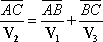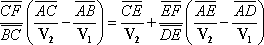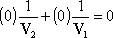## Solution to the 4 Travelers problem

Miguel Branchtein, Brasil
July 27, 2003

Here's another solution to the 4 travelers' problem.

We draw the four straight lines where travelers 1, 2, 3 and 4 respectively travel. Their meeting points are A, B, C, D, E and F.

Given that they meet in A, B, C, D and E, we must show that they also meet in F.

The straight line FG is just an auxiliary line, drawn from F paralel to line 1. The point where it crosses line 2, we'll call it G.We callthe distance between points A and B, and so forth. We call V1 the speed of traveler 1, and so forth.

From triangle ABC, we see that, in order that travelers 2 and 3 meet in point C, in the same time traveler 2 goes from A to C, traveler 1 must go from A to B, and then traveler 3 goes from B to C. That is,

 (1)Similarly, from triangle ADE, we have

 (2)At last, from triangle CEF, we get

 (3)Given (1) and (2), we have to prove (3).

We substitute 1/V3, from (1), and 1/V4 , from (2), into (3).Putting together all terms in 1/V1 and 1/V2

 (4)In order to evaluate these, we observe that triangle GFC is similar to triangle ABC:And triangle GFE is similar to triangle ADE:Substituting these relations in (4), we getorproving eq. (4), and consequently eq. (3).